#Function Repository Resource:

# EulerEquations

Get the Euler–Lagrange differential equations derived from a given functional

Contributed by: Wolfram Research
 ResourceFunction["EulerEquations"][f,u[x],x] returns the Euler–Lagrange differential equation obeyed by u[x] derived from the functional f, where f depends on the function u[x] and its derivatives, as well as the independent variable x. ResourceFunction["EulerEquations"][f,u[x,y,…],{x,y,…}] returns the Euler–Lagrange differential equation obeyed by u[x,y,…]. ResourceFunction["EulerEquations"][f,{u[x,y,…],v[x,y,…],…},{x,y,…}] returns a list of Euler–Lagrange differential equations obeyed by u[x,y,…],v[x,y,…],….

## Examples

### Basic Examples

The Euler equations for the arc length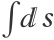in two dimensions yields a straight line:

 In:=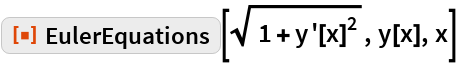Out=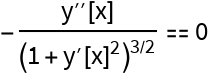In:=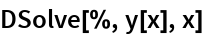Out=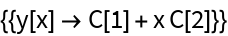A simple pendulum has the Lagrangian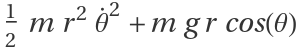:

 In:=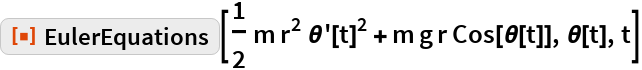Out=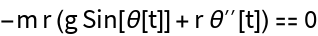The solution to the pendulum equation can be expressed using the function JacobiAmplitude:

 In:=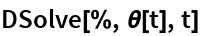Out=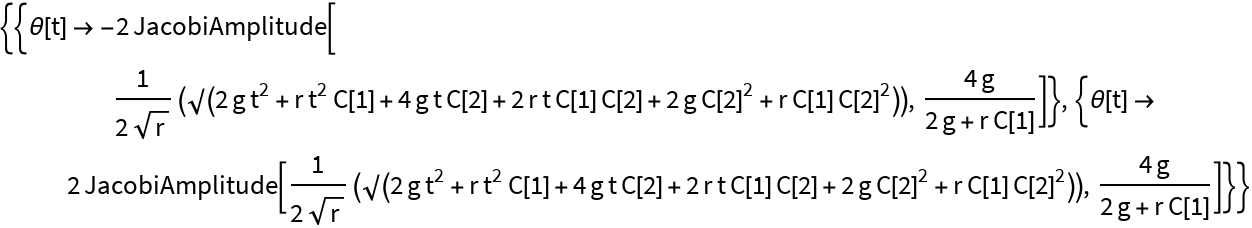### Scope

The Lagrangian of a point particle in two dimensions has two dependent variables and yields Newton's equations:

 In:=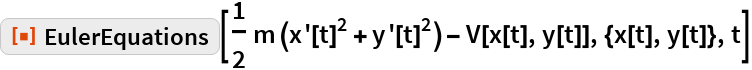Out=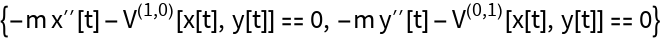The Lagrangian of a point particle in two dimensions with a central potential:

 In:=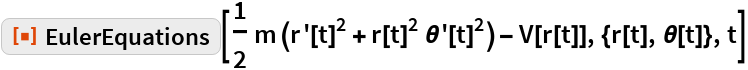Out=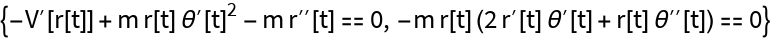Second- and higher-order derivatives may be included in the integrand. A Lagrangian for motion on a spring using higher-order terms:

 In:=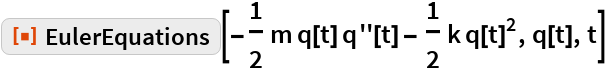Out=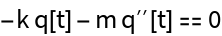The integrand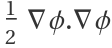has several independent variables:

 In:=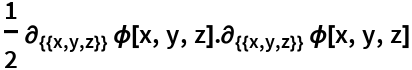Out=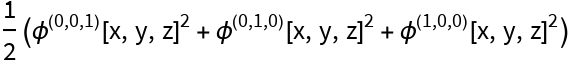The Euler equations yield Laplace's equation:

 In:=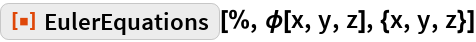Out=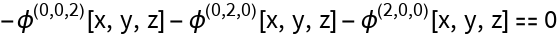### Applications

The Euler equations for the integrand f[yxx,yx,y,x]:

 In:=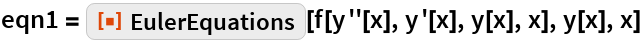Out=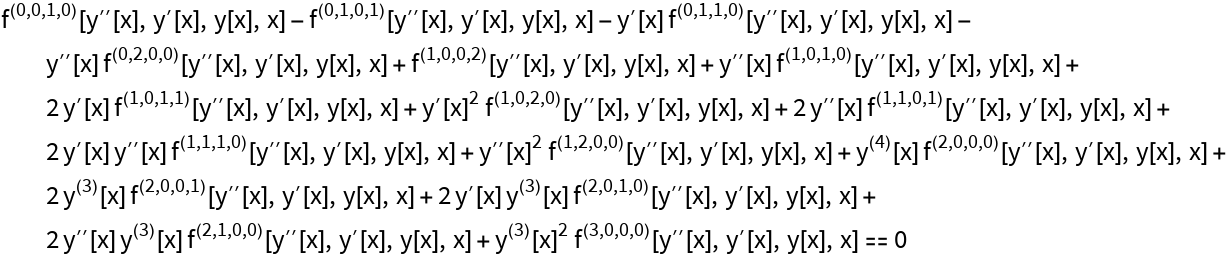In:=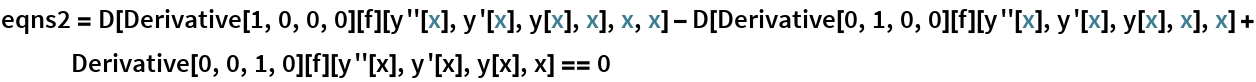Out=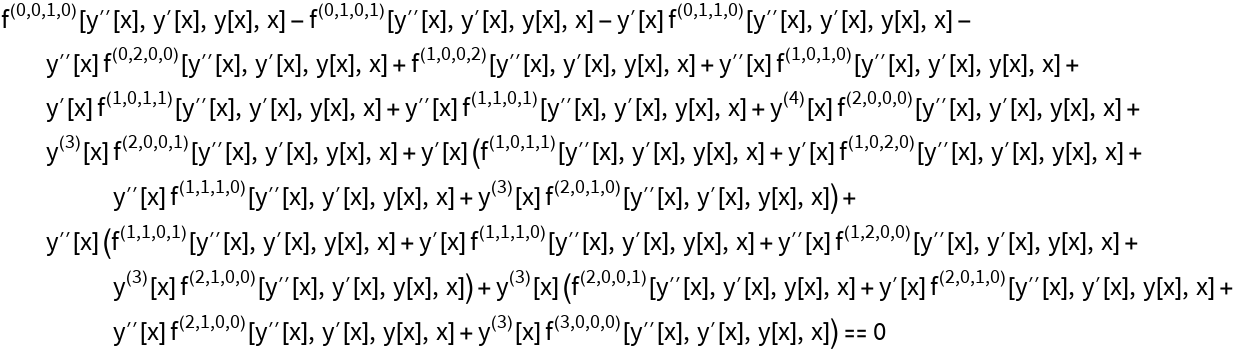Check:

 In:=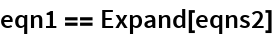Out=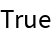The brachistochrone problem asks for the curve of quickest descent. The time taken for a particle to fall an arc length ds is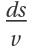. If y measures the decrease in height from an initial point of release, then the velocity v satisfies:

 In:=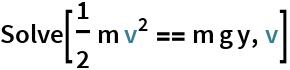Out=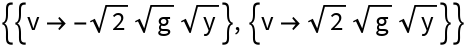The equation for a curve joining two points, where a particle starting at rest from the higher point takes the least amount of time to reach the lower point:

 In:=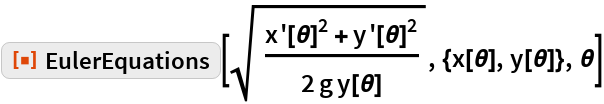Out=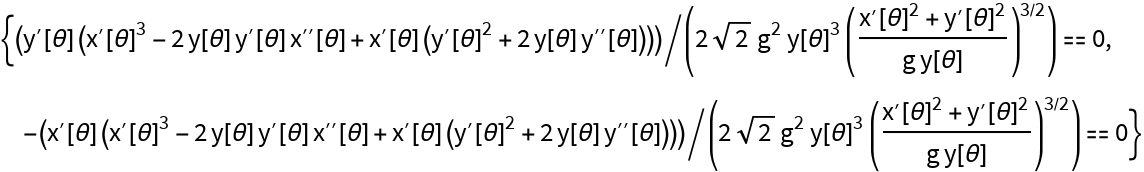It is well known that the solution to the brachistochrone problem is a cycloid:

 In:=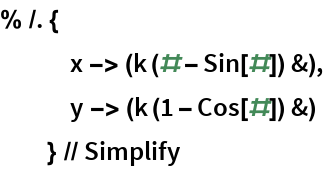Out=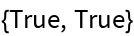The Lagrangian for a vibrating string yields the classical wave equation:

 In:=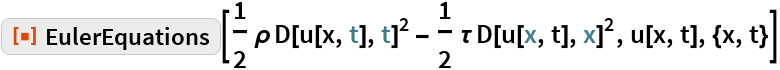Out=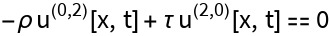## Requirements

Wolfram Language 11.3 (March 2018) or above